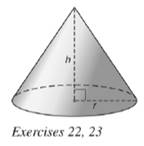Chapter 9.3, Problem 22EElementary Geometry For College St...

7th Edition
Alexander + 2 others
ISBN: 9781337614085

Solutions

Chapter
SectionElementary Geometry For College St...

7th Edition
Alexander + 2 others
ISBN: 9781337614085
Textbook Problem

For the accompanying right circular cone, h = 6 m and r = 4 m. Find the exact and approximatea) lateral area.b) total area.c) volume.To determine

(a)

To find: The exact and approximate lateral area of the right circular cone.

Explanation

Given:

Consider a right circular cone with h=6 m and r=4 m.

Formula used:

In a right circular cone r2+h2=l2.

The lateral area of the right circular cone is L=πrl

Calculation:

We have h=6 m and r=4 m.

Substitute the known values in the formula,

r2+h2=l242+62=l216+36=l2<

To determine

(b)

To find: The exact and approximate total area of the right circular cone.

To determine

(c)

To find: The exact and approximate volume of the right circular cone.

Still sussing out bartleby?

Check out a sample textbook solution.

See a sample solution

The Solution to Your Study Problems

Bartleby provides explanations to thousands of textbook problems written by our experts, many with advanced degrees!

Get Started

Evaluate the integral. 13. tcsc2tdt

Calculus: Early Transcendentals

Fill in each blank: 56floz=pt

Elementary Technical Mathematics

Find the coordinates of the points that are 5 units away from the origin and have an x-coordinate equal to 3.

Applied Calculus for the Managerial, Life, and Social Sciences: A Brief Approach

Draw a polygon for the distribution of scores shown in the following table. X f 6 2 5 5 4 3 3 2 2 1

Essentials of Statistics for The Behavioral Sciences (MindTap Course List)

Evaluate the integral, if it exists. x+2x2+4xdx

Single Variable Calculus: Early Transcendentals